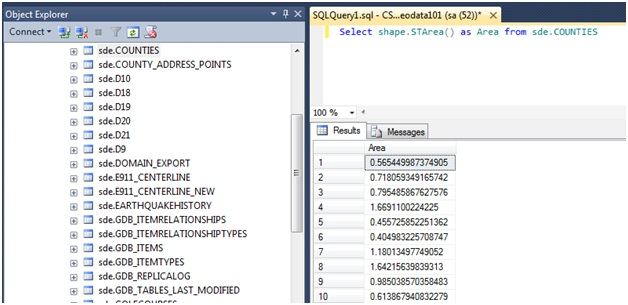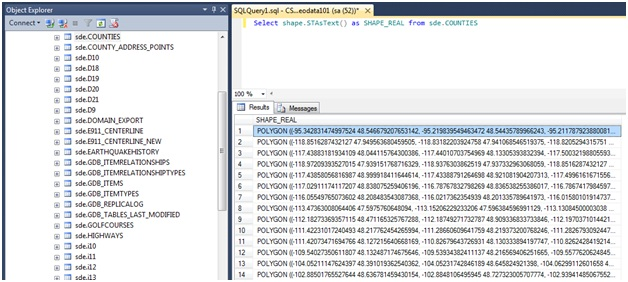HOW TO

# Calculate the area of data stored using either the SQL Geometry or SQL Geography data type

## Summary

The instructions provided can be used to calculate the total surface area of data stored using either the SQL Geography or the SQL Geometry data type in a SQL Server 2008 or SQL Server 2012 database. The syntax provided is executed using a query in SQL Server Management Studio (SSMS).

When used with the SQL Geography data type, the results of the STArea() are returned in squared units of the linear measure defined by the spatial reference system of the geography instance. When used with the SQL Geometry data type, the results of the STArea() are the squared units in which the coordinates were supplied.

So, if using a coordinate system where coordinate values are measured in meters, the area is measured in meters squared. If the coordinates were supplied in feet, the area is in feet squared.

## Procedure

• Execute the following syntax to capture the total surface area of a feature class stored using the SQL Geometry data type within SSMS.

`> Select shape.STArea() as Area from sde.COUNTIES`

`Note:Substitute the feature class to be measured for the one listed above as sde.COUNTIES.`• Execute the following syntax to capture a more in-depth query that displays all of the individual vertex coordinates values for each feature.

`> Select shape.STAsText() as SHAPE_REAL from sde.COUNTIES`Article ID:000011702

Software:
• Legacy Products

Get help from ArcGIS experts

Contact technical support